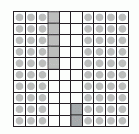Name: ___________________Date:___________________

 Email us to get an instant 20% discount on highly effective K-12 Math & English kwizNET Programs!

### Grade 5 - Mathematics Decimal Sums

 Write the decimals one below the other. Note: The point must be aligned and the numbers one below the other. Example: Add 0.35 and 0.42 Consider a square being divided into 100 equal parts.Shade 3 tenths and colour five hundreths which shows 0.35. Shade 4 tenths and colour 2 hundreths which shows 0.42. Count the total number of tenths and hundreths in the square.Answer: 0.77 Directions: Add the following decimal numbers. Also write at least ten examples of your own.
 Question 1: 927.76 + 642.65

 Question 2: 631.90 + 480.72

 Question 3: 6732.9 + 345.2

 Question 4: 7.3909 + 7.3886

 Question 5: 24.841 + 5.948

 Question 6: 5.634 + 15.851

 Question 7: 1.2440 + 8.7662

 Question 8: 84.513 + 70.427

 Question 9: 47.826 + 57.898

 Question 10: 4571.0 + 7645.3

 Question 11: 21.506 + 69.229

 Question 12: 6493.1 + 3242.0

 Question 13: 392.9 + 9875.0

 Question 14: 2.4738 + 7.2660

 Question 15: 235.6 + 9650.1

 Question 16: 7460.0 + 213.5

 Question 17: 3115.2 + 1357.6

 Question 18: 1.6304 + 9.7089

 Question 19: 738.77 + 449.16

 Question 20: 2489.3 + 8962.6

 Question 21: 3.5617 + 1.1132

 Question 22: 1060.6 + 7506.4

 Question 23: 3266.8 + 7799.3

 Question 24: 5.7182 + 0.2732

 Question 25: 5.5583 + 0.4088

Question 26: This question is available to subscribers only!

Question 27: This question is available to subscribers only!

Question 28: This question is available to subscribers only!

Question 29: This question is available to subscribers only!

Question 30: This question is available to subscribers only!